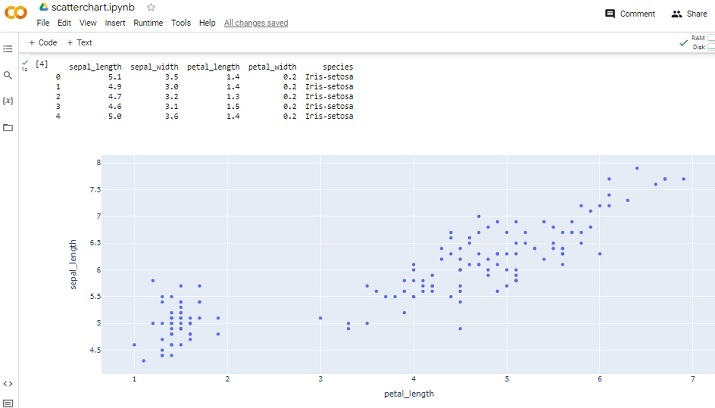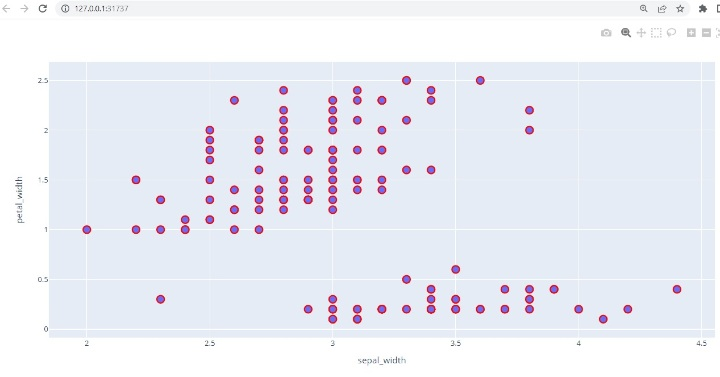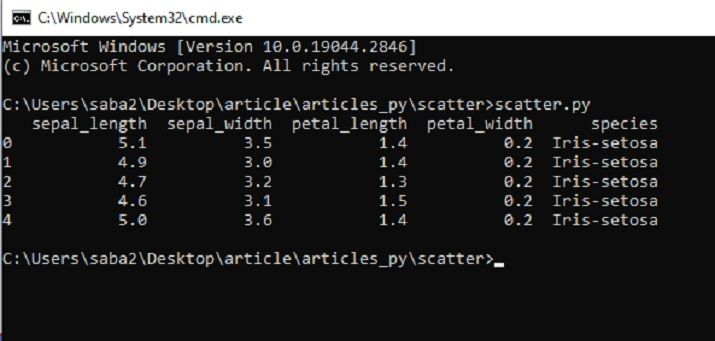# How to make a basic Scatterplot using Python-Plotly?

Sometimes, the task is to analyze a dataset and use charts or plots for data visualization. Plotly is a nice open-source graphing library that can be used with Python and is used for making a variety of plots and charts quickly and easily. In this article, using two different examples, this Python library called Plotly is used with Python code to make the scatter plots. In the first example, the Python installed in the computer system is used to run a Python program that is written for making a scatter plot. In another example, using the Google Colab the method is shown where without having Python installed in the computer, Python and Plotly can still be used and a scatter plot can be made. In both these examples, the open-source dataset from Kaggle is used for the data analysis and visualizations.

## The IRIS.csv file Used

sepal_length,sepal_width,petal_length,petal_width,species
5.1,3.5,1.4,0.2,Iris-setosa
4.9,3,1.4,0.2,Iris-setosa
4.7,3.2,1.3,0.2,Iris-setosa
4.6,3.1,1.5,0.2,Iris-setosa
5,3.6,1.4,0.2,Iris-setosa
5.4,3.9,1.7,0.4,Iris-setosa
4.6,3.4,1.4,0.3,Iris-setosa
5,3.4,1.5,0.2,Iris-setosa
4.4,2.9,1.4,0.2,Iris-setosa
…….., ….., ……, ……., ……..


This CSV file contains five columns named sepal_length, sepal_width, petal_length, petal_width, and species. Out of these, we will use sepal_width, and petal_width for the scatter plot in Example 1 and we will use sepal_length, and petal_length for the scatter plot in Example 2.

## Example 1: Making the Scatter Plot Using Python and Plotly

### Design Steps and Coding

• Step 1 − First import pandas and plotly. Plotly is the open-source graphing library for Python that will be used for making scatter plots.

• Step 2 − Now read the IRIS.csv file as the dataset given here will be used for making the scatter plot.

• Step 3 − Make a dataframe dff and show the columns and content of this dataframe.

• Step 4 − Make a scatter plot, using the scatter() function, and specify the sepal_width for x axis and petal_width on y axis.

• Step 5 − Set the style of the marker such as size and color.

• Step 6 − Write the function to show the scatterplot. Run the program using the cmd window. The plot will open in a new tab in the browser.

## Example 2: Making the Scatter Plot Using Python and Plotly on Google Colab

### Design Steps and Coding

• Step 1 − Login using Google account. Go to Google Colab. Open a new Colab Notebook and write the Python code in it.

• Step 2 − Upload the IRIS.csv file that was downloaded from Kaggle and saved, using the link given in the example 1, as the dataset given here will be used for making the scatter plot.

• Step 3 − Now import pandas and plotly. Plotly is the open-source graphing library for Python that will be used for making scatter plots.

• Step 4 − Make a dataframe dff and show the columns and content of this dataframe.

• Step 5 − Make a scatter plot, using the scatter() function, and specify the petal_length for x axis and sepal_length on y axis.

• Step 6 − Write the function to show the scatterplot. Run the program by clicking the playbotton on the given code cell. Check the result as it will be displayed in the colab notebook.

## Example 1: Making the Scatter Plot Using Python and Plotly

### Saving the data File / csv File Required for data Analysis

To make the scatter plot, we will use the data available on Kaggle. Login to Kaggle and download the CSV file from this link −

Make a file Called Scatter.py. Write the Following Code in This File

#include the required libraries
import pandas as pd

#This library is needed to make the scatter plot
import plotly.express as pxx

#read the CSV file and make a dataframe

#print the columns and data

#make the scatter plot
figg = pxx.scatter(dff, x="sepal_width", y="petal_width")

#set the properties of the scatter plot
figg.update_traces(marker=dict(size=12, line=dict(width=2, color="red")), selector=dict(mode='markers'))

#display the chart
figg.show()


### Run the Python file in the Command Window## Viewing The Result - Example 1## Example 1: Making the Scatter Plot Using Python on Google Colab

#Uploading the csv


### Including the Libraries and Reading the CSV file

import pandas as pdd
import plotly.express as pxx



### Printing the Result and Showing the Scatter Plot

print(dff.head())
figg = pxx.scatter(dff, x="petal_length", y="sepal_length")
figg.show()


### Viewing The ResultIn this Python and Plotly article, by two different examples, the ways to show how to make a scatter plot using the Python library called Plotly are given. First, the method is given where the dataset from Kaggle is downloaded and saved for analysis. Then a Python program is written to make a scatter plot using the functions from Plotly. In the second example, Google Colab is used for writing the Python program and making the scatter plot using the same library and the same data set.

Updated on: 04-May-2023

255 Views